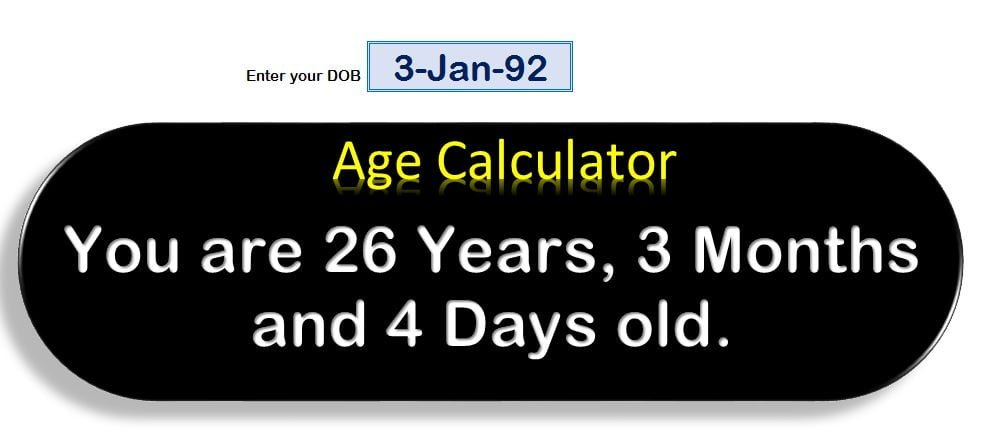Home>Templates>Age Calculator in Excel

# Age Calculator in Excel

Tallying the number of years that have passed since a person’s date of birth is a time-consuming and convoluted task, particularly when multiple individuals are involved. But have no fear, the DATEDIF function is here to make the process of calculating age a breeze. In this thought-provoking post, we delve into the intricacies of creating an age calculator in Excel using the DATEDIF formula. Get ready to uncover the mystery behind determining the exact age, in years, months, and days, until the present date.

## The Enigma of the DATEDIF Function:

The DATEDIF function is a built-in function in Excel that computes the difference between two dates. It returns the difference in an interval specified by the user – years, months, or days – based on the interval argument. The function requires three arguments: the start date, the end date, and the interval argument, which specifies the type of difference desired. For instance, “Y” represents years, “M” represents months, “D” represents days, and so on.

### Calculating Age in Excel: A Formulaic Approach

To calculate age in years, months, and days, we will harness the power of the DATEDIF function three times. The first formula calculates the number of years between the date of birth and the current date. The second formula calculates the number of months between the date of birth and the current date, including the number of years. The third formula calculates the number of days between the date of birth and the current date, including both the number of years and months. The formulas are as follows:

Years: “=DATEDIF(A2,TODAY(),”Y”)”
Months: “=DATEDIF(A2,TODAY(),”YM”)”
Days: “=DATEDIF(A2,TODAY(),”MD”)”

To provide the age in a single, concise sentence, we will use an additional formula that combines the above three formulas. The formula is as follows: “=You are “&N1&” Years, “&N2&” Months and “&N3& ” Days old.”

## Using the Age Calculator Template:

The Age Calculator template is a pre-constructed spreadsheet that simplifies the process of finding your age. To utilize it, follow these steps:

• Open the Age Calculator template.
• Enter your date of birth in the DOB box.
• The age calculator will automatically compute your age in years, months, and days.
• The result will show your age in a single, easy-to-understand sentence, such as “You are 26 Years, 3 Months, and 4 Days old.”Age Calculator in ExcelIn conclusion, the Age Calculator template is a straightforward and user-friendly tool that assists in calculating age in Excel. The DATEDIF function makes the process of calculating age an effortless task. This template is designed for accuracy and can be used for personal or professional purposes. By following the steps outlined above, you can find your age in no time. Get ready to unravel the perplexities of age calculation with this template.

Watch the video tutorial for how to create Age Calculator in Excel